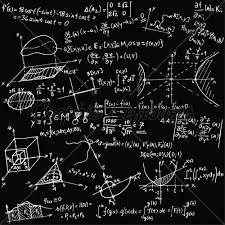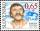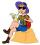# Equation 15

Solve equation with variables on both sides:

x =  -24

### Step-by-step explanation:

1/2x+2=3/8x-1

1/2•x+2=3/8•x-1

x = -24

Our simple equation calculator calculates it.Did you find an error or inaccuracy? Feel free to write us. Thank you!Tips to related online calculators
Need help to calculate sum, simplify or multiply fractions? Try our fraction calculator.
Do you have a linear equation or system of equations and looking for its solution? Or do you have a quadratic equation?

## Related math problems and questions:

• 15 numberWhat number is smaller (greater) by 15 than its half?
• How many 15How many six-eights are there in 18? Which of the following would you choose to solve this question? 18 x 6/8 or 18 : 6/8
• ExpressionIf it is true that (l + 15 w)/(w) = 6, then the value of the expression (13 l)/(12 w) is:
• To improper fractionChange mixed number to improper fraction a) 1 2/15 b) -2 15/17
• Equation 11Solve equation: 0=y-1,2.(y-1,5)
• Land costThe land is worth 33295 CZK. 1st owner has 5/15, 2nd owner has 4/15, 3rd owner has 1/5 4th owner has 1/5. How much CZK each of them will receive?
• Simple equation 324 = n • 27, solve for n
• Find xSolve: if 2(x-1)=14, then x= (solve an equation with one unknown)
• EquationSolve equation and check the result: 1.4x - 3/2 + x - 9,8 = x + 0,4/3 - 7 + 1,6/6
• Reciprocal equation 3Solve reciprocal equation: 1/2 + 2/3=1/x
• Equivalent fractionsAre these two fractions equivalent -4/9 and 11/15?
• HikerHiker went half of the trip the first day, a third of the trip the second day, and remains 15 km. How long the trip he planned?
• Simple equation 5Solve equation with fractions: X × 3/8 = 1/2
• LunchLunch is given to seniors from 12:15 to 12:40 during the Coronavirus pandemic. What angle will the minute hand of clock describe during this time?
• Simplest form of a fractionWhich one of the following fraction after reducing in simplest form is not equal to 3/2? a) 15/20 b) 12/8 c) 27/18 d) 6/4
• Equation 25Solve following simple equation: 3/4(x+5)=1/2(x+9)
• Cakes1/3 poppy cake, 1/3 apple, 15 pieces of cheese. How many are totally cakes?